• Slides: 29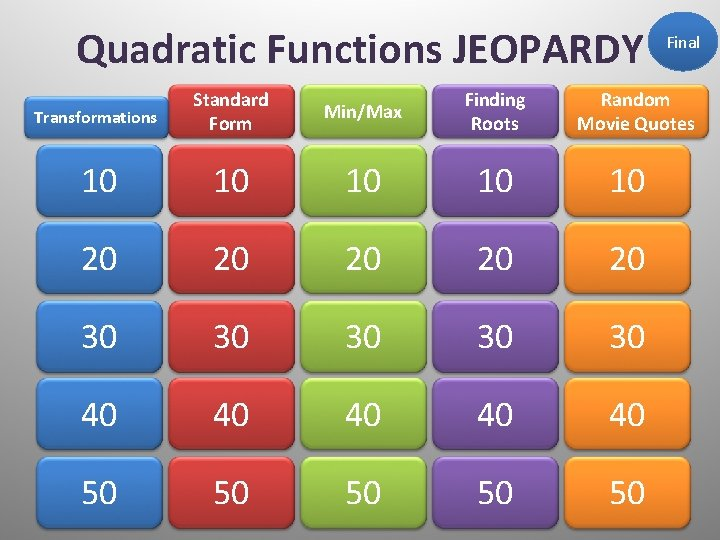Quadratic Functions JEOPARDY Transformations Standard Form Min/Max Finding Roots 10 10 10 20 20 20 30 30 30 40 40 40 50 50 50 Final Random Movie QuotesGraph the following: 10 points Answer:Graph the following: 20 points Answer: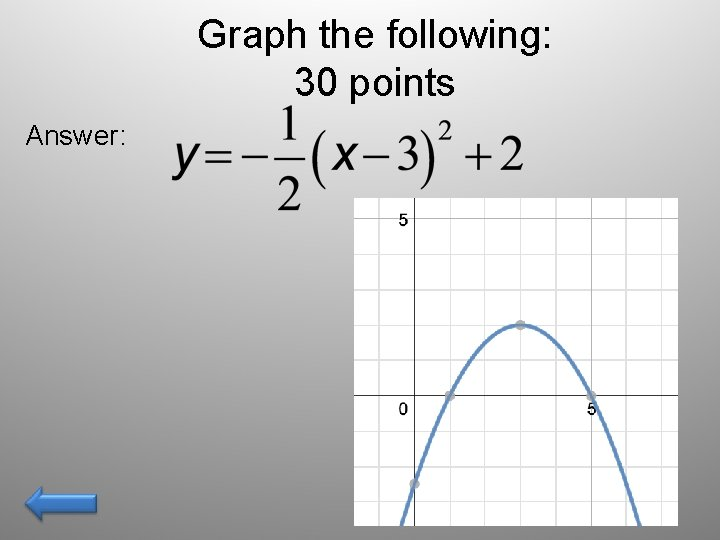Graph the following: 30 points Answer:What is the equation of the following: 40 points Answer:What is the equation of the following: 50 points Answer:What is the vertex of the following: – 10 Points Answer: (4, 1)What is the axis of symmetry for the following: – 20 Points Answer: X=1Find the axis of symmetry for the following– 30 Points Answer: X=4Find the axis of symmetry for the following: – 40 Points Answer: X=2Answer the following: – 50 Points • An object is launched at 19. 6 meters per second (m/s) from a 58. 8 -meter tall platform. The equation for the object's height s at time t seconds after launch is s(t) = – 4. 9 t 2 + 19. 6 t + 58. 8, where s is in meters. When will the object reach its max height and what will the height be? Answer: 2 seconds 78. 4 meters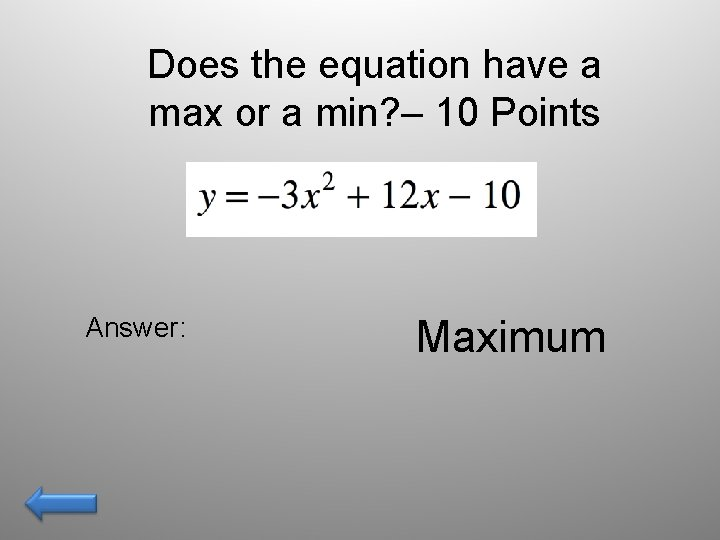Does the equation have a max or a min? – 10 Points Answer: MaximumDoes the graph have a max or min and what is the value? – 20 Points Answer: Maximum, 0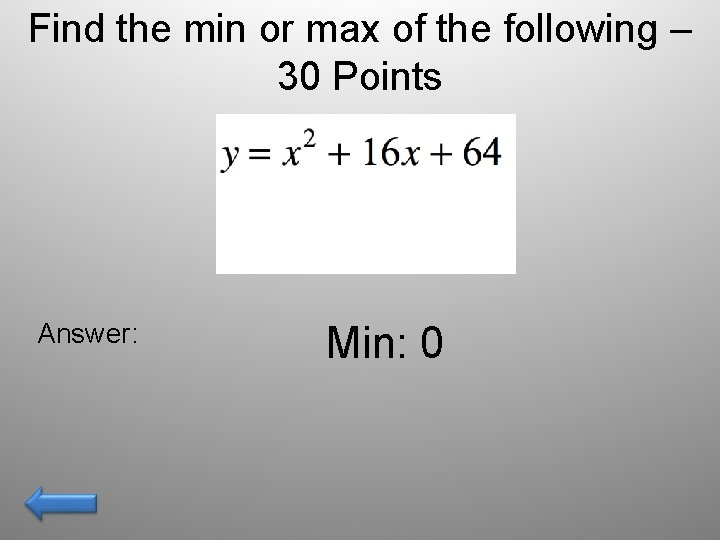Find the min or max of the following – 30 Points Answer: Min: 0Find the min or max of the following equation – 40 Points Answer: Vertex: -3, -1 Max: -1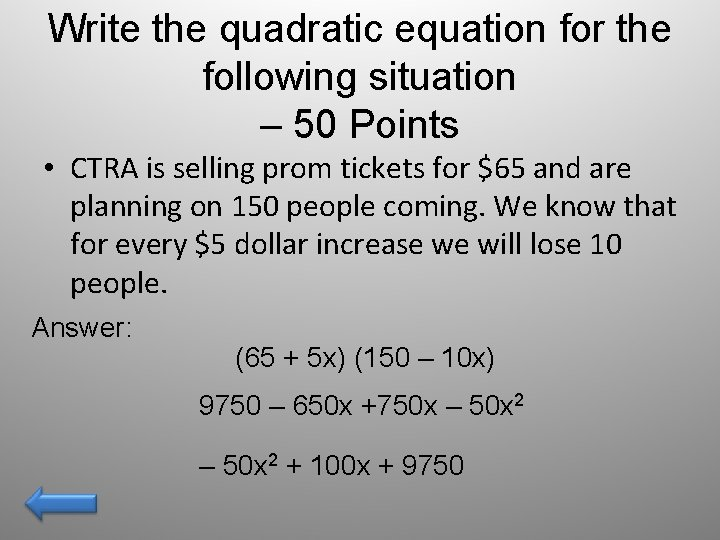Write the quadratic equation for the following situation – 50 Points • CTRA is selling prom tickets for \$65 and are planning on 150 people coming. We know that for every \$5 dollar increase we will lose 10 people. Answer: (65 + 5 x) (150 – 10 x) 9750 – 650 x +750 x – 50 x 2 + 100 x + 9750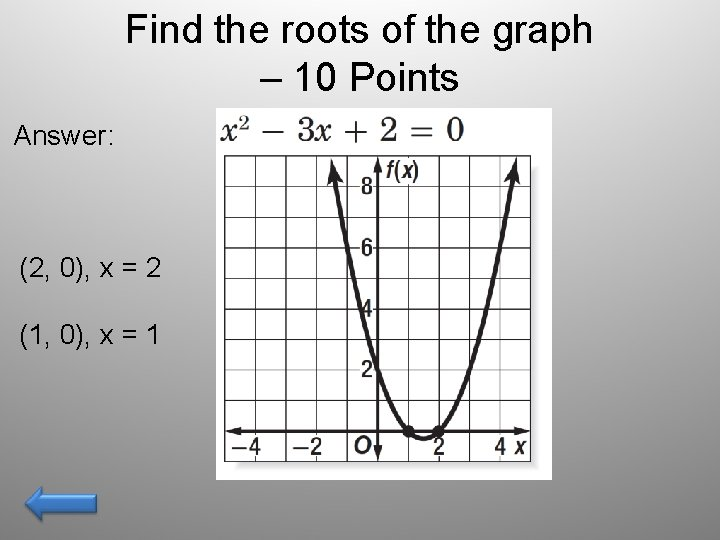Find the roots of the graph – 10 Points Answer: (2, 0), x = 2 (1, 0), x = 1Estimate the Roots of the Graph – 20 Points Answer: Around: (. 9, 0), x =. 9 (4. 1, 0), x = 4. 1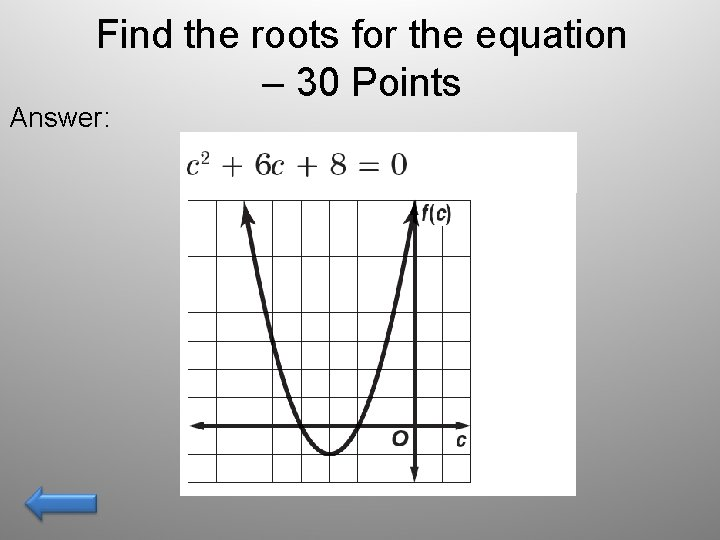Find the roots for the equation – 30 Points Answer: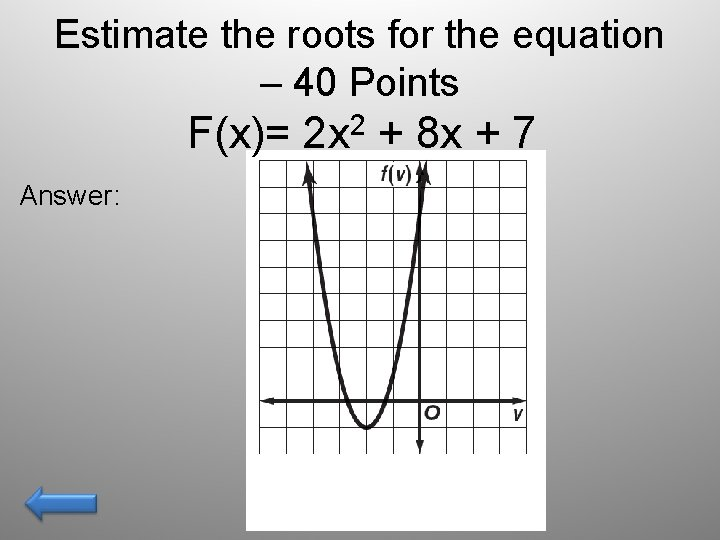Estimate the roots for the equation – 40 Points F(x)= Answer: 2 2 x + 8 x + 7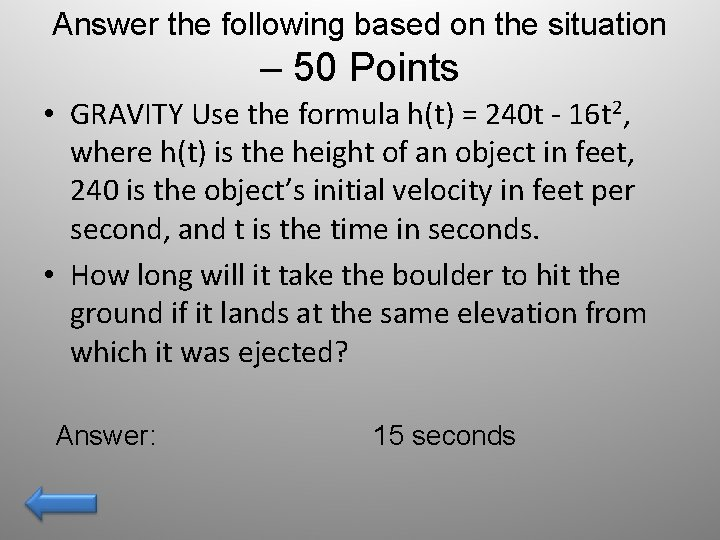Answer the following based on the situation – 50 Points • GRAVITY Use the formula h(t) = 240 t - 16 t 2, where h(t) is the height of an object in feet, 240 is the object’s initial velocity in feet per second, and t is the time in seconds. • How long will it take the boulder to hit the ground if it lands at the same elevation from which it was ejected? Answer: 15 secondsName the Movie– 10 Points • • • Hakuna Matata; what a wonderfull phrase. Hakuna Matata; It aint no passing craze. It means no worries, for the rest of your days. Its our problem free, philosophy. . . HAKUNA MATATA Answer: Lion KingName the Movie– 20 Points • We are going to need a bigger boat!! Answer: JawsName the movie– 30 Points • Do or do not, there is no try. Answer: Star Wars, Empire Strikes Back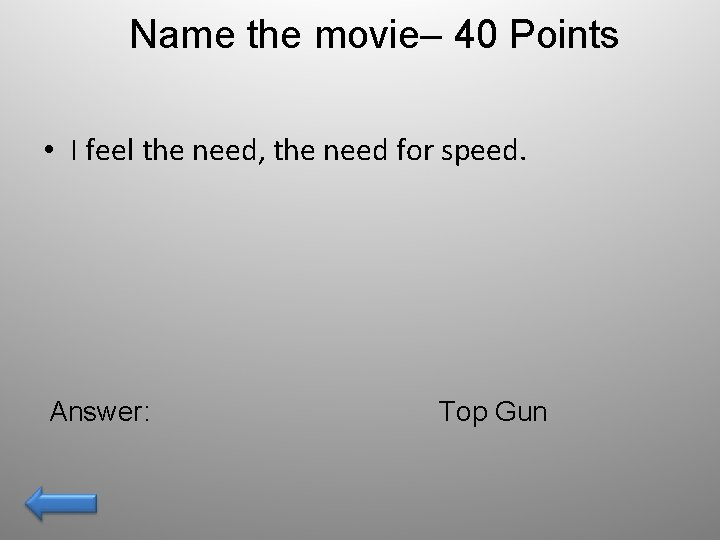Name the movie– 40 Points • I feel the need, the need for speed. Answer: Top GunName the Movie– 50 Points Would you trade all the days, from this day to that, for one chance, just one chance, to come back here and tell our enemies that they may take our lives, but they will never take our FREEDOM!!! Answer: BraveheartFINAL JEOPARDYSOFTBALL OLYMPIC SOFTBALL GOLD MEDALIST MICHELE SMITH PITCHES A CURVEBALL WITH A SPEED OF 64 FEET PER SECOND. IF SHE THROWS THE BALL STRAIGHT UPWARD AT THIS SPEED, THE BALL’S HEIGHT H IN FEET AFTER T SECONDS IS GIVEN BY H = -16 T 2 + 64 T. FIND THE COORDINATES OF THE VERTEX OF THE GRAPH OF THE BALL’S HEIGHT AND INTERPRET ITS MEANING.